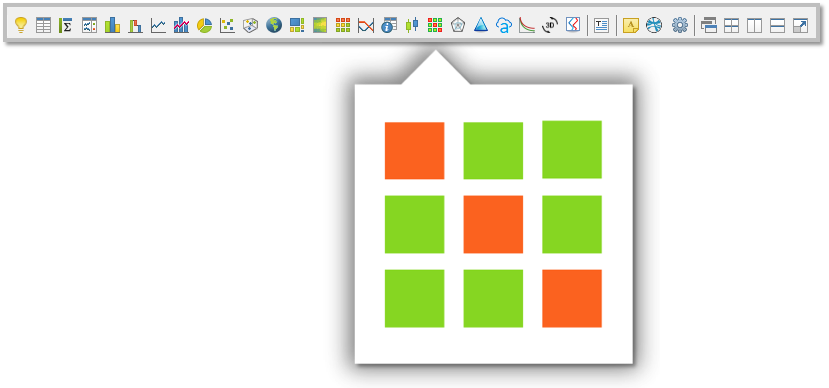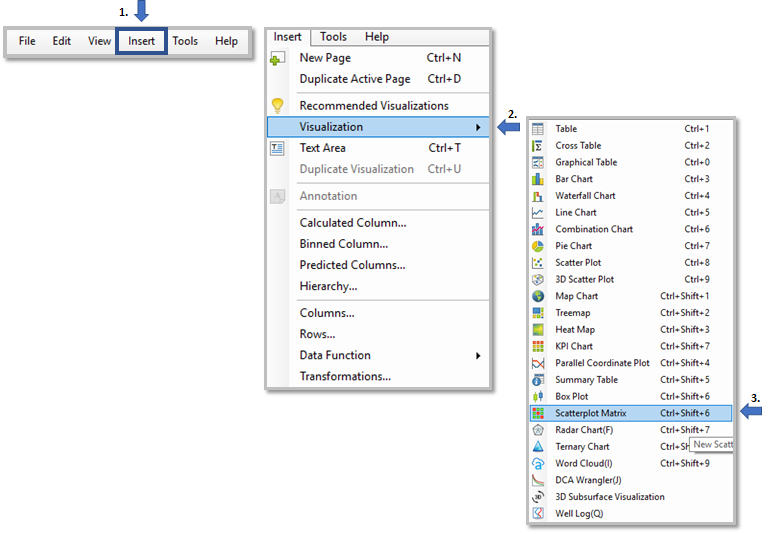## What is the RAI Scatterplot Matrix?

Scatterplot Matrices are a great way to roughly determine if you have a linear correlation between multiple variables. This is particularly helpful in pinpointing specific variables that might have a similar correlation. Data is divided into groups with each groups containing a specified number of traits. These traits are displayed in a matrix format so that traits can be compared. Along the diagonal, there are histograms for each trait. Above the cells of the histogram are the labels for the traits. Above the cells of the scatterplots is the Pearson Coefficient Score.

### Purpose

Use the scatterplot matrix extension to understand the relationship between parameters. This visualization creates a scatter plot per each combination of variable allowing got identify correlation.

#### Where is the Scatterplot Matrix Visualization?#### Another way to access Scatterplot Matrix visualization

1. Click "Insert" on the top menu bar.
2. Go to "Visualization
3. Click Scatterplot Matrix.#### Outputs:

• One Scatterplot Matrix Visualization
• Histogram (diagonal) - Traits
• Scatterplot - Correlation between the multiple variables.
•  (Above each Scatterplot is a Pearson Coefficient Score)

Example: * This Image was taken from "Scatter Plot Matrix Example" template from the Exchange.ai Portal.Link for the template example from Exchange.ai Portal

Scatter Plot Matrix Template Example